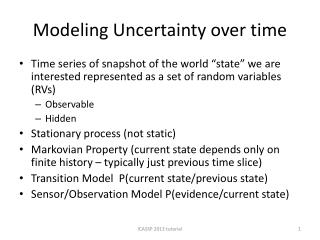DownloadDownload PresentationModeling Uncertainty over time

# Modeling Uncertainty over time

Download Presentation## Modeling Uncertainty over time

- - - - - - - - - - - - - - - - - - - - - - - - - - - E N D - - - - - - - - - - - - - - - - - - - - - - - - - - -
##### Presentation Transcript

1. Modeling Uncertainty over time • Time series of snapshot of the world “state” we are interested represented as a set of random variables (RVs) • Observable • Hidden • Stationary process (not static) • MarkovianProperty (current state depends only on finite history – typically just previous time slice) • Transition Model P(current state/previous state) • Sensor/Observation Model P(evidence/current state) ICASSP 2013 tutorial

2. Inference tasks in temporal models • Filtering: posterior distribution over current state given evidence = likelihood of evidence • Prediction: posterior distribution of future state given evidence to date • Smoothing: posterior distribution of past state given all evidence up to the present • Most likely explanation: given sequence of observations, most likely sequence of states that has generated them • EM-algorithm • Estimate what transitions occurred and what states generated the sensor reading and update models • Updated models provide new estimates and process iterated until convergence ICASSP 2013 tutorial

3. Uncertainty and Time Hidden Markov Models I p( | ) Emission Probs t MODEL Model Transition Probs P( | ) t t-1 Observations Hidden 1 2 3 4 Hidden State (single discrete variable) Observed ICASSP 2013 tutorial

4. Uncertainty and Time Kalman Filtering • Streams of noisy input data • Basic idea t->t+1 : • Prior knowledge of state • Prediction step (based on some model) • Update step (compare prediction to measurements) • Readjust model • Output estimate of state • Statistically optimal estimate of system state • Particle filters are another approach ICASSP 2013 tutorial

5. Kalman Filter • Linear Gaussian conditional distributions represent state and sensor models • LG: P(x/y)=N(ay y + by, σy)(c) • Next state is linear function of current state plus some Gaussian noise i.e constant dx/dt • Forward step: mean + covariance matrix at t produces mean + covariance matrix at t+1 • Trade-off between observation reliability and model reliability • Variants to relax strong assumptions: switching, extended ICASSP 2013 tutorial